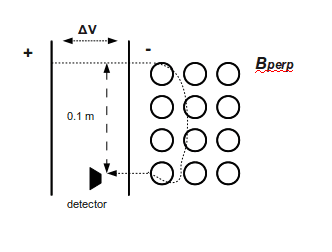# Mass spec voltage and magnetic field

sweetdion

## Homework Statement

These question deal with a mass spectrometer whose slits are separated by .1 m.

a) Trying to calibrate it using protons, for B=.2T, the proton starting at rest should be accelerated through a voltage of _________ to have it pass through the two slits.

b) To set it with He2+ ions, with 2 protons and 2 neutrons, so that they pass through both slits, they need a voltage of _________. (Take into account a fixed geometry where r and B are constant.

c) Assuming that the earth's magnetic field ifs 0.5x10^-4 and is perpendicular to path of particles, how much error is possible using the He2+ particles?

1/2mv^2=qV
F = qvB
qE = qvB -->

## The Attempt at a Solution

a) should i use the 3rd equation for this one?
b) same equation but change value for q to 2q?
c) I have no idea on this one

## Answers and Replies

Homework Helper
Can you show a picture? I can not imagine those slits.

ehild

sweetdion
Can you show a picture? I can not imagine those slits.

ehildthis is the best i could do. The line should be curvy but i did it by freehand and the B circles should have dots in the center of them

sweetdion
been working on this a bit more. Some new equations have caught my eye.

v=E/B, mv^2=qV so v=squareroot(2qV/m)

R=mv/qB
F=-qvBkhat
q/m=E^2/2VB^2

I think the last equation is the best one, but I dont know how to find the electric field.

Homework Helper
So the trajectory of the particle must be a half-circle between the two slits. OK.

To calculate the speed of the particle you need the voltage V between the electrodes it traverses (you denoted it by delta V but it is difficult to write that delta). As you know, the electric field does qV work, that work increases the kinetic energy so

1/2 mv2= qV (eq. 1)

You have written this equation in your first post.

You know that the Lorentz force on a particle in a magnetic field is

FL=qvB

when the v velocity of the particle is perpendicular to the field lines.

This force drives the particle around a circle of radius R, so the Lorentz force is equal to the centripetal force. The centripetal force is

Fcp=mv2/R.

FL=Fcp ----> mv2/R=qvB,

solving for v, it is

v=qBR/m (eq. 2).

The particle makes a half circle, so the distance between the slits is the diameter of the circle, how long is then R?

Now, about the calibration. The particle is proton. What are the mass and charge of the proton? You find them anywhere.

You know R, m, q, B, calculate the speed of the proton, then use eq. 1 to get the voltage between the electrodes.

Now do the same with the alpha particle.

ehild

sweetdion
okay but with the alpha particle, to calculate the velocity do i just add up the charges and the masses? when I add up the charges...they cancel, and I get 0. I don't think the velocity can be 0.

sweetdion
nevermind...i consulted wikipedia and found the answer to that question. thanks for your help! Now to find the error using the alpha particles. I plug in the new B and see what I get? Or should I add B of earth and 0.2T. Then compare what I get from using this B with the first B? But the Earth's electric field is perpendicular, how does that enter into the equation...

Last edited:
Homework Helper
nevermind...i consulted wikipedia and found the answer to that question. thanks for your help! Now to find the error using the alpha particles. I plug in the new B and see what I get? Or should I add B of earth and 0.2T. Then compare what I get from using this B with the first B? But the Earth's electric field is perpendicular, how does that enter into the equation...

Add the contribution of Earth to the original B and see the difference you get in the voltage. Then do the same with subtracting the field of Earth, as you do not know, if the magnetic fields point in the same or opposite directions. Take the deviation of the voltages from the original voltage and choose the magnitude of the bigger one as the uncertainty. Or maybe, you need to give it in percent of the original voltage.

ehild

sweetdion
thanks so much on this problem ehild.:-)

sweetdion
edit: nvm this post